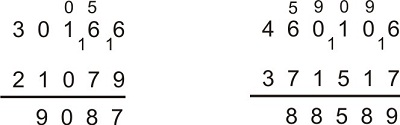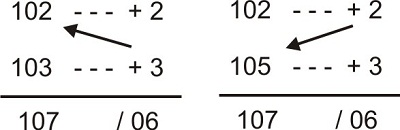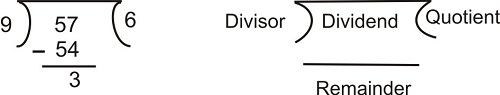# Speed Calculation Techniques

Since from our childhood we learnt mathematics in such a way that most of us really dread maths when they come to engineering.

1.   How to additions fast. For example we have to do a simple addition like 234 + 563+ 985 + 349.

Alternative Method 1:

Instead of doing like this we first add all the digits in the hundred's place.  2 + 5 + 9 + 3 = 19. Put 2 zero's to the right hand side so we get 1900.
Now add the digits in the tenth's place. 3 + 6 + 8 + 4 = 21. Put 1 zero to the right hand side so we get 210.
Now add the digits in the units place 4 + 3 + 5 + 9 = 21.
Now 1900 + 210 + 21 = 2131

Alternative Method  2:
First add all the hundreds to the first number. So we get 234 + 500 + 900 + 300 = 1934.  Now add the tens to this number 1934 + 60 + 80 + 40 = 1934 + 180 = 1934 + (200 - 20 ) = 2134 - 20 = 2114.
Now add the unit digits to this number = 2114 + 3 + 5 + 9 = 2014 + 17 = 2114 + ( 20 - 3 ) = 2134 - 3 - 2131.
If you do adequate practice you can easily add numbers without much effort. Only thing required is practice.

Subtractions:
Subtractions are simple when you remember only two things.
When you are subtracting a bigger digit from a smaller digit, you have to borrow 1 from the digit left to it. Then the left digit gets reduced by 1. If the left most digit is 0, then we have to borrow from the digit left to it.  Now the 0 should  assume as 9.  If the digit to the left is 1, then it becomes 0. Observe the following two examples.  Digit borrowed has written the the left bottom and changed digit has written in small digit on top of the existing digit.Multiplications:
If you remember multiplication tables up to 12 th table, this is enough.
We know that (a + b) $\times$ c = ac + bc.  Using this rule simplifies our calculations a lot.

2. Find 12 $\times$ 19
Sol. Mentally imagine this number as (10 + 2 ) $\times$ 19 = 190 + 38 = 228.

3. Find 24 $\times$ 16
Sol. Above expression can be written as  (20 + 4) $\times$ 16 = 320 + 64 = 384

4. Find 103 $\times$ 105 =
Sol. By taking 100 as a base we can easily get the answer for the above question within seconds.
103 is 3 more than 100 and 105 is 5 more than 100 So we will write

Add the excess of the second number to first number or excess of first number to second number as show below  to get left most digits and multiply the excesses to get the right most two digits.Note: Always remember if you get a single digit number put zero in the left two make it a two digit number to get the right most two digits.

5. Find 102 $\times$ 103 =
Sol.If the product on the right hand side is more than 100  let us say 378 add 3 to the digit in the hundred's place.
We have to find 118 $\times$ 121
Now this 3 should be added to 139 So our final answer = (139 + 2) / 78 = 14178

6. Find 95 $\times$ 98
Sol. Here both numbers are less than 100. So they are deficient of - 5 and - 2 compared with 100. So

7. Find 92 $\times$ 106
Sol.Now reduce 1 from the hundred's place and add 100 to rightmost two digits.
So our answer is (98 - 1) / (100 - 48 ) = 9752

Similarly we can multiply two digit numbers with base 10. Here we should write only one digit after the slash and the carry over should be added to left most digits.
For example 12 $\times$ 18 = (12 + 8)/16 = 216.
19 $\times$ 14 = (19 + 4 ) /36 = 266

Criss Cross Method:

If the numbers are not greater than 20 then this method is not so useful.  So we use another interesting method called "Criss Cross method".

8. Find 23 $\times$ 46
Sol.Step 1 : 2 $\times$ 4 = 8
Step 2 : (2 $\times$ 6 + 3 $\times$ 4) = 24
Step 3 : 3 $\times$ 8 = 18
We have to write the above numbers in a specific format $8{}^24{}^18 = 1058$  .  Here we added the super scripts to the digits lie to the left of them.

9. Find 76 $\times$ 95 =
Sol.
Step 1 : 7 $\times$ 9 = 63
Step 2 : (7 $\times$ 5 + 6 $\times$ 9) = 89
Step 3 : 6 $\times$ 5 = 30
$63{\rm{ }}{}^89{\rm{ }}{}^30 = 7220$

If this addition is a bit confusing you can write the numbers as below.  First write 63.  Then for 89 write 8 on top of 3 and write 9 beside 8. For 30 write 3 on top of 9 and write 0 beside 9. Now add normally

10. 345 $\times$ 763 =
Sol. As this questions involves 3 digit numbers we have to follow the following approach.

Step 1 = 3 $\times$ 7 = 21
Step 2 = 3 $\times$ 6 + 4 $\times$ 7 = 44
Step 3 = 3 $\times$ 3 + 4 $\times$ 6 + 5 $\times$ 7 = 68
Step 4 = 4 $\times$ 3 + 6 $\times$ 5 = 42
Step 5 = 5 $\times$ 3 = 15

11. 342 $\times$ 76
Sol. Here we assume 76 as 076 and follows the above procedure.

As  has zero at the leftmost place, the first step results in zero. So we simply omit all the steps which involve 0.
Step 1 = 0
Step 2 = 3 $\times$ 7 = 21
Step 3 = 3 $\times$ 6 + 4 $\times$ 7 = 46
Step 4 = 4 $\times$ 6 + 7 $\times$ 2 = 38
Step 5 = 2 $\times$ 6 = 12

Division and Divisibility :

Suppose, we divide 57 by 9 asThen, 9 is the divisor, 57 is the dividend, 6 is the quotient and 3 is the remainder.
Therefore, Dividend = (Divisor × Quotient) + Remainder.

12. Find the remainder when 10,000 is divided by 84
Sol.Important Technique to find quotient or percentages:

13. Find $\displaystyle\frac{{3486}}{{189}}$
Sol. First we make the denominator 200. i.e., we are introducing an error of 11 which is $\displaystyle\frac{{11}}{{189}} = \displaystyle\frac{1}{{17}}$ th of the denominator.
Now $\displaystyle\frac{{3486}}{{200}} = 17.43$
But this answer is less than actual answer. So we have to increase it by 1/17th part of it.
$\displaystyle\frac{1}{{17}} \times 17.43 \simeq 1$

So final answer = 17.43 + 1 = 18.43

14. Find $\displaystyle\frac{{283}}{{1983}} \times 100$
a. 127%
b. 14.3%
c. 13.6%
d. 15.1%
Sol. Never start finding common factors.
Adjust the denominator to 2000. So we are introducing an error of 17 which is $\displaystyle\frac{{17}}{{1983}} = \displaystyle\frac{1}{{117}}$ th of the denominator.
$\displaystyle\frac{{28300}}{{2000}} = 14.15$

Now $\displaystyle\frac{{14.15}}{{117}}$ = 0.12 approximately.
Adding 0.12 to 14.25 gives 14.27.
Option B is correct.

Calculating Percentages:

Suppose we are asked to find 55 % of 123. As per the formula we have to calculate $\displaystyle\frac{{55}}{{100}} \times 123$which involves big calculations.

So remember the following. (x + y + z...)% (K) = x%(K) + y%(K) + z%(K)...
Now if abcde is a number,
10% (abcde) = abcd.e (divide the given number by 10)
1% (abcde) = abc.de (divide the given number by 100)
0.1% (abcde) = ab.cde (divide the given number by 1000)
......
50% (abcde) = half of the given number
So
50% (123) = 61.5 (divide the given number by 2)
5% (123) = 6.15 (simply divide 50% by 10 to get 5%)
55% (123) = 67.65

Finding Squares:

Suppose we want to find the square of 86.  We can use the above techniques like cris-cross method to find the square of this number as 86 + (-14) / 196 = 7396

But we can also find the square by using this number by using this formula $100 + 2d/{d^2}$.  Here d is how much the given number is greater or less than 100. If the given number is less than hundred, d is negative otherwise positive. Here d = - 14

So ${86^2}$= 100 - 2 $\times$ 14 /196 = 7396.

Always remember, whenever you take 100 as a base we should put only 2 digits right side of the slash. The excess should be carried over.

15. ${105^2}$=
Sol. Here d = 5.
100 + 2 $\times$ 5 / 25 = 11025

16. ${995^2}$=
Sol. Here d = 5, Base is 1000 and there should be three places after the slash
1000 - 2 $\times$ 5 / 0025 = 990025

17. ${9996^2}$=
Sol. Here d = -4.
But we have to take the base as 10000. Also the number of the digits after slash should be 4.
10000 - 2 $\times$ 4 / 0016 = 99920016

Note: If any number is not near to 100 or a multiple of 100 then use "Criss Cross method " to find the square.

Finding Cube roots:

If d is the difference between the given number and 100 multiple near to that number then cube of a given number = $100 + 3d / 3{d^2}/{d^3}$

18. Find ${105^3}$
Sol. Here d = 5 and two digits should be there between and after the slashes.
100 + 3 $\times$ 5 / 3 $\times$ 25 / 125 = 115 /75/125 = 1157625

19. Find ${96^3}$
Sol. Here d = -4
$100 + 3 \times - 4/3 \times - {4^2}/ - {4^3}$ = 88 / 48 / -64 = 88 / 47 / (-64 + 100) = 884736

Finding cube roots of TWO digit numbers:

To find cube root of the given number first we have to remember the cubes of first 10 natural numbers.
${1^3} = 1$,${3^3} = 27$,${4^3} = 64$,${5^3} = 125$,${6^3} = 216$,${7^3} = 343$,${8^3} = 512$ ....
One interesting observation is, for 1, 4, 5, 6, 9 we get the same unit digit.  And for the remaining digits the sum of the given number and units digits of its cube is 10.
2 + 8 = 10, 3 + 7 = 10, 7 + 3 = 10, 8 + 2 = 10.

20. Find the cube root of 185193.
Sol. Observe unit digit here is 3 so unit digit of the answer is 7.  Now omit 193. Now find after which perfect cube 193 lies. It is 125.  So our tenth place is 5. So answer is 57.

21.   Find the cube root of 804357.
Sol. Unit digit of the answer is 3. (cube of 3 = 27). Now omit 357 and find after which cube 804 lies. It is 729. so our tenth place is 9.

Other Application of Vedic Mathematics:

Digit sum Rule**:
Digit sum is the sum of the digits of the given number until we get a single digit number. This digit sum rule applies for addition, subtraction and multiplication.

For example,  56 $\times$ 8 = 448.
Digit sum of 56 = 5 + 6 = 11 = 2
So 2 $\times$ 8 = 16 = 1 + 6 = 7.
Now observe 448 = 4 + 4 + 8 = 16 = 1 + 6 = 7.

22. What is the value of 39856 $\times$ 139?
a. 5839984
b. 5539984
c. 5839981
d. 5529984
Sol. First we will calculate digit sums.
39856 = 3 + 9 + 8 + 5 + 6 = 31 = 4
139 = 1 + 3 + 9 = 13 = 4. So the answer will have a digit sum of 4 $\times$ 4 = 16, i.e. 7. Only the digit of options (b) and (c) is 7. However, we also know the answer should end in 4 as product of the unit digits 6 and 9 = 54.  So the correct answer is (b).